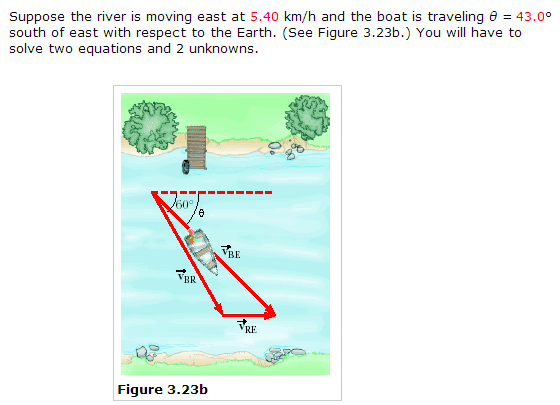Relative Motion problem that doesn't have right triangle relationship

Homework Statementa) Find the speed of the boat with respect to the Earth. (km/h)

b) Find the speed of the boat with respect to the river if the boat's heading in the water is 60° south east (km/h)

Homework Equations

v(x) = v cos(θ)
v(y) = v sin(θ)
v = √(vx^2+vy^2) but can't use it in here because it is not a right trangle :(
θ = tan^-1 (vy/vx)

The Attempt at a Solution

I would have easily solved this problem if only I had one unknown. I am completely lost. I barely have any idea on what I am doing. I would love to hear an explanation of each step because I really want to know how to do these sort of problems when the formed triangle isn't a right triangle and when there are two unknowns.

What I have so far is that
V of the boat with respect to the river = V br
V of the boat with respect to the earth = V be
V of river with respect to the earth = V re

V be = V br + V re

V br (x) = ? sin(43) ; V br (y) = ? cos(43)
V be (x) = ? ; V be (y) = ?
V re (x) = 5.4 ; V re (y) = 0

We've never learned this but after researching on the solution I found out how to solve relative motion problems that don't have a right triangle with ijk vector component addition. Another method was also through Law of Sines and Cosines. What I came up with was:

ijk method:
V be = V br + V re
V re = 5.4i; V br = cos(60)i + V br sin(60)j; Vbe = V be cos (43)i + V be sin (43)j

Law of Sines and Cosines method:
V be^2 = V br^2 + V re^2 - 2 * V br * V re * cos 163

This might be incorrect since I barely have any idea about what I am doing. Anyways, even with just plugin it in I still don't know how I can I simplify it to solve it since I still have 2 unknowns.

How can I solve this problem, somebody help D:

ehild
Homework Helper
You know that the velocities are vectors and add together by components. Taking the positive x axis pointing to East, and the y axis pointing to South, the velocity of the boat with respect to the river is V =Vcos(60°)i + Vsin(60°)j, and the velocity of the river is Vr=5.4 i, the velocity of the boat with respect to the ground Vg=V+Vr =Vgcos(43°)i+Vgssin(43°)j. (bold means vectors)

Compare both the x and y components of the vector sum. You get two equations for the unknowns V and Vg.

ehild

can you explain a little bit further in, I've never done anything like this, we didn't study ijk method at all in class before. I feel really stupid, but none of this really make sense to me

NascentOxygen
Staff Emeritus
ijk method:
V be = V br + V re
V re = 5.4i
V br = cos(60)i + V br sin(60)j; Vbe = V be cos (43)i + V be sin (43)j
You wrote: V be = V br + V re
re-arranging, gives V br = V be - V re

Use this relation to determine Vbr components:
i component: Vbe cos 43° - 5.4
j component: Vbe sin 43°

and it is given that tan 60° = (j component) ÷ (i component)
leaving you with one equation in one unknown, solve for Vbe

NascentOxygen
Staff Emeritus
The question as presented seems a bit misleading. You can't find a unique solution to (a) without needing the information on the 60° path contained in (b).

ehild
Homework Helper
can you explain a little bit further in, I've never done anything like this, we didn't study ijk method at all in class before. I feel really stupid, but none of this really make sense to me

Have you solved such problems concerning vector addition with the Cosine and Sine Laws?

Yo can find the angles of the shaded triangle in the picture.

ehild